GRAVITY
(July, 1998)

By Noel Huntley, Ph.D.

There is little doubt that gravity presents us with a puzzling and somewhat abstruse subject; in other words, it is a difficult one. In fact gravity could be considered to be an enigma. It would be perfectly possible to have a successful technology of antigravity spacecrafts (control over gravity) but still not properly understand gravity.

That gravitational theory is more complex than is generally appreciated is partly due to the fact that one can obtain 3D interpretations which appear to be satisfactory and complete, but they will be found to be dependent on a 4D understanding. At the 3D level, one may derive a particle or radiation interpretation, but 4th dimensionally the holistic nature can be understood, of the unitary oscillation of, say, the planet, which gives rise to, or reduces to, the 3D and limited explanation. A particular difficulty is that gravity needs to be compatible with quantum theory and thus must possess quantum attributes itself but at the same time it must reduce to the special case of Newton's laws.

Gravity appears to have a single pole; whereas we know from electricity and magnetism that an opposite pole is required to bring about attraction (or repulsion). Also mass only attracts (apparently) mass; it doesn't repel in our known universe.

One can immediately draw certain theoretical tentative conclusions from a scenario in which an object appears to be operating as a single pole (gravity) but its constituents are also involved in two-polarity systems (electricity and magnetism). We can know from 4D geometry that an oscillation can be at right angles to 3D space; compared with electromagnetic oscillations which act across or within our 3D space.

Imagine a taut elastic diaphragm in which vibrations are created by plucking it 1) along its plane, or 2) at right angles to the plane. That is, creating oscillations along the plane or at right angles. We are presenting here a 2-to-3 dimensional analogy, but representing 3-to-4 dimensions. We shall see that gravity is related to the oscillations at right angles, not along the plane of the diaphragm (that is, 3D space).

In the 4D system only one pole is apparent or detectable in 3D; the other is along a line at right angles to each of the 3D axes, that is, in a 4D direction---this corresponds to the example of a direction at right angles to the elastic diaphragm. The poles for gravity are above and below the diaphragm (or either one in the plane) but for the EM example the poles are in the plane. With a 3D mechanism both poles are apparent in 3D space, for example, north and south pole of a magnet. However, in the 4D system only one pole can exist at a time in 3D.

We might at this point query as to why the gravity system has attraction instead of repulsion (equally probable) since in electricity and magnetism we have both. The reason is: there is no attraction. We might mention here that in Newtonian physics it was necessary to have a mysterious attraction concept but this was handled in Einstein's general relativity by space geometry/curvature, though it ignored the polarity problem, and relativity does not give the machinery behind the curvature.

We have covered in previous articles some of the details of the 4D vortex theory. A mass forms at the centre of its vortex. Mass does not cause space to 'pucker', that is, create curvature, as in general relativity. The mass comes into existence simultaneously with the space around it. The vortex is a centripetal spiral transducing energy from 4D to 3D. The centre of the vortex, for example, the mass, is in 3D. The periphery of the vortex is in 4D or higher.

These basic vibrations create spacetime and mass---there is a spiral oscillation from the periphery of the vortex to its centre and back. This is a little like imagining space around an object contracting inwards, imploding, and 'condensing' into mass. This implosive, centripetal spiral generates mass. On the opposite cycle the centrifugal action---expanding outwards---dissipates mass and creates space. This action is both 4D and 3D. It cuts across 3D (that is, in the 4D direction) but causes waves along 3D.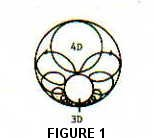Thus if one is considering a planet and its gravity, one can envisage an implosive pressure, basically from a 4D configuration into 3D---see Figure 1. This pushes particles and objects (as described in the article on inertia) towards the centre of the planet. But what about the part of the oscillation cycle in which the pressure is not only released but is 'moving' in the opposite direction, as antigravity does. We may see that antigravity is the opposite side of the gravity wave---like the opposite side of a coin with both faces identical. For example, the negative of the wave is the same as the positive but turned around (mirror image). Note that in Figure 1 the 3rd dimension is shown outside the 4D circle---it should be in the centre but is difficult to illustrate adequately. The actual shape of the energy is a 4D toroid (doughnut) but one can visualise a 3D toroid---however, this 4D visualisation is not essential for this article. See Figure 5.

At this point let us ask why is there apparent attraction between masses, or more realistically, why do they move together? The answer is that this is a natural product of overlapping vortices. Imagine a hollow sphere with energy, say, light energy (for simplicity and a better pictorial demonstration) reflecting from the inside towards the centre where the mass or planet is. Figure 2 refers.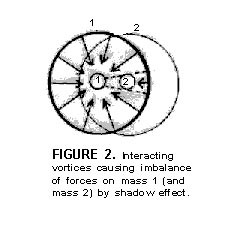If we now place a second mass in such proximity to the first so that it is partially inside the 'sphere' of the first, then we can also see that the first mass is inside the sphere of the second mass.In Figure 2 we can see that for mass 1 the energy is partially blocked by mass 2---in fact mass 2 casts a 'shadow' on mass 1. Since the arrows represent pressure then we can see that there is a net pressure from left to right, causing mass 1 to move towards mass 2. And of course vice versa.  This pressure is provided by the centripetal action of the vortex. There is a potential difference between the vortex periphery and its centre.

Now interference from all the vortices in the universe---the planet's vortex, the stars, galaxies, etc.---will create countless nodes as described in the article on superspace and inertia. These nodes provide a 3D expression of the implosive pressure to pin objects to the surface, etc. There is confusion in current physics regarding the relationship between the inertial force, the gravitational force, and the gravitational field.

The gravitational field effect, as described in the article on inertia, is quite different from the inertial force and gravitational force. The principle of equivalence in general relativity applies to the inertial and gravitational force. However, there is not actually any principle of equivalence because these two forces are identical; that is, they are the same. Gravitational nodes passing through a body resting on the Earth's surface are constantly dragging it downwards as one set of resonating nodes after another try to lock onto the nodes within the body. This is the same effect as a body out in space which is being pulled in any direction, causing acceleration of the body and the dragging of the body's nodes away from spacetime nodes, which are attempting to lock on by resonance. This is described in the article on inertia. This is the Newtonian force. However, there is no Newtonian force when the body falls in a gravitational field; it is carried by and resonates with the spacetime nodes.

So far, we have only described the effects of the vortex which expresses gravity in a 3rd-dimensional sense. That is, the nodes, which we may call gravitons, press towards the centre of the planet and pin objects to the surface. This is the gravitational force, and is identical to the inertial force. The vortex is an oscillation. It is a centripetal energy spiralling from a 4th-dimensional to a 3rd-dimensional configuration. This is now attempt to explain this more basic property.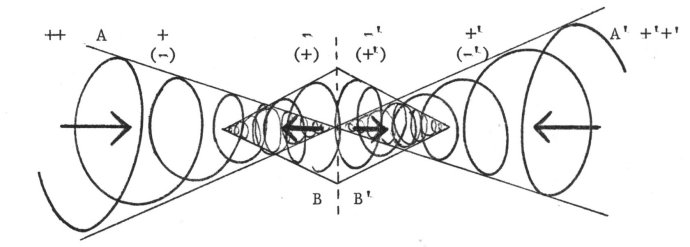FIGURE 3

One can see from the diagrams in the article on the basic energy unit that there are two spirals from the 4D periphery which meet in the centre of the vortex. This is illustrated differently here in Figure 3 where the ends A and A' are not shown coming from the same source (4D) for convenience of illustration.

The important point to realise is that only one side of the oscillation appears in our 3D space, that is, one pole---see Figure 3. The other pole, or flow, is antigravity relative to our gravity (they are both gravity in opposition).

From Figure 3 we see that there are polarities A to A', a 4D oscillation, but within this are A to B and A' to B' which are 4D-to-3D oscillations. These latter two would correspond to Dr Russell's centrifugal components (the energy is coming out into 3D). Thus on the left of the figure, say, our universe side, we have the 4D, A - A' flow which is at right angles to our 3D. Within this w e have A - B, a 4D-to-3D flow providing the 3D gravity aspect of particles pressured towards the centre of the Earth. The same applies mirror-imagewise on the anti-universe side. However, only the 4D flow cuts all the way across from A to A'. Thus the 3D anti-flow of particles from the anti-universe don't affect our side. Nevertheless the 4D comes in on the anti-cycle into our universe side---at 90 degrees to 3D.

It is only the 4D aspect which comes through on the anti-cycle and it affects the particles in our space differently now. Instead of creating changing potential in space as does the gravity half cycle, via gradient 4D-3D, it influences the particles to have the same potential across space---this can be called waving in time. It thus gives a rectification of the particles. These gravitons pressure towards the centre of the Earth on the gravity half cycle but don't reverse on the anti-half cycle, since the spatial effect on them has changed to temporal. There is a reciprocation of time and space---see Figure 4. This is a theory which has at least the potential to explain what is happening with these complex and hidden processes.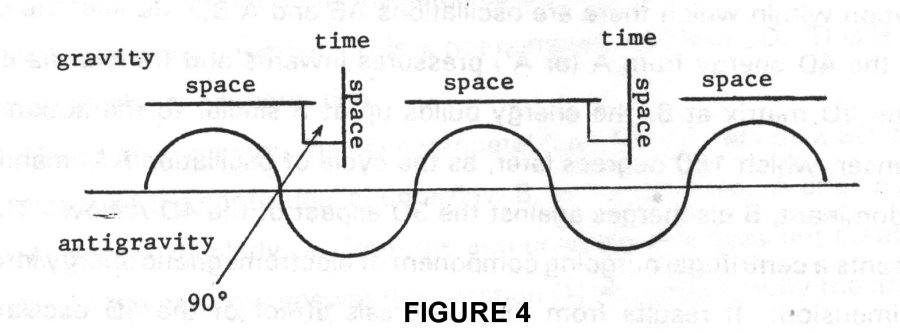Continuing with this theory, and at the risk of repetition, Figure 3, as inferred, is another view of the same configuration as Figure 1, except that A and A' are not brought together and emanating from the same 4D source. The extra vortices in the middle are due to an inductance that occurs in 3D due to this oscillating 4D gravity/antigravity wave. This is a transformer-like action which creates a hysteresis effect. Without this hysteresis effect there would be no 3D universe as we know it. What this effect does is to create not only separate 3D vortices B and B' (on each side) but ones which are in a different phase state with their originating 4D vortex. There is a hysteresis lag of the 3D electromagnetism B and B' induced by the gravity/antigravity oscillation. The phase state of such 3D vortices would be expected to vary for different vortices. Thus compared with Figure 1, Figure 3 now includes the separate 3D secondary vortices.

As the 4D energy from A (or A') pressures inwards and transforms down into the 3D matrix at B/B', the energy builds up at B/B' similar to the action of a condenser, in which 180 degrees later, as the cycle of oscillation AA' manifests A as dominant, B discharges into our 3D in this 4D A-flow. Thus B represents a centrifugal outgoing component of electromagnetic energy into the 3rd dimension. It results from the hysteresis effect of the 4D oscillations spiralling into 3D---the fundamental oscillations experience a dragging effect similar to the effect of electrons oscillating in an antenna causing the fields to interfere and propagate outwards producing EM waves. Now this happens on both sides of the centripetal oscillations, and would be expected to occur with all continuous or standing-wave vortices. Figure 5 shows the secondary vortex from a 3D (more realistic) viewpoint.

Figure 3 shows the fundamental and dynamic effect of what causes gravity in isolation. On the left side A represents the 4D centripetal gravity flow in our 3D. On the right, in the anti-universe side we have an identical situation but in reverse, that is, mirror image. This nevertheless gives us a potential for antigravity on our side since it is basically all superimposed. The difficult geometry to visualise is that A and A' are from the same source---see Figure 1---but each opposite side with respect to the other is 'inside' the other, not extended along linear 3D space (see article on basic energy unit). The centripetal spiral A pressures in and by hysteresis and inductance creates the central electromagnetic centrifugal spiral---the radiation cycle of Dr. Russell's configuration---and expands outwards into our 3D.

We might note that we have two 4D poles within which two 3D poles (on each side) are formed. This could be referred to as a four-polarity system. This configuration would apply to all natural entities from atoms to planets, stars, galaxies, etc. with a resultant infinite interference effect creating a myriad miniblackholes and miniwhiteholes of spacetime.

Some physicists have concluded that space has both positive and negative miniblackholes and miniwhiteholes. That is, four types: a negative ingoing blackhole and a positive ingoing blackhole, and similarly for the whiteholes. This can have some meaning when we consider that the gravity field has a hierarchy from atoms to planets, stars, galaxies, etc. all superimposed and interferring, creating infinite minute vortices/nodes/gravitons of space. With this in mind, now imagine this topology structure incorporating the above four-polarity system, giving four different 'hole' attributes (this paragraph can be skipped).

In summary, the primary 4D gravity/antigravity vortex due to hysteresis would be expected to create a secondary out-of-phase radiation vortex. The gravity component and the radiation component form our 3D space (and the antigravity and its radiation component form the space on the anti-universe side). The 4D antigravity potential on our side is homogeneous spatially but varies temporally and is not normally detected, nor does it subtract from our gravity. Similarly for the other side.

Thus the primary gravity vortex induces a secondary electromagnetic vortex with lines of electromagnetic force that are at right angles to the centripetal spiral (lines). These primary and secondary vortices interfere to produce a consistent grid system around different entities. The points of interference create criss-crossing lines of force, forming nodes in repeated patterns of motion (for example, orbits) for establishing, say, electrons in atoms, or large nodes around the Sun for carrying planets. See Figure 5.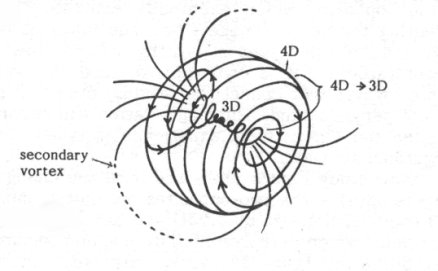FIGURE 5

Gravity thus appears to have a dynamic and static phase. The dynamic action is the oscillation caused by the macro-polarity, 4D to 3D, pressuring centripetally into the 3rd dimension. The static phase is the effect of all the minute interference nodes and gravitons passing through objects as they are pressured implosively (by the dynamic phase) towards the centre of the body, such as Earth.